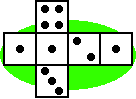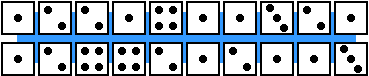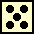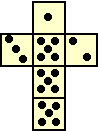Objective 13 Distinguish between experimental and theoretical probability for single events.
Problem
 Jessica rolled her die 20 times and kept track of the values rolled in a list. A net for Jessia's die is shown. What is the theoretical probability of rolling a one? What is the experimental probability of rolling a one?Manipulative
Materials: event cards, overhead spinner, spinner mat (1-4), regular six-sided die, 4 beans, paper cup, coin, paper bag (containing 5 blue, 3 red and 2 yellow tiles), deck of cards (A to 10 in one suit).

 Draw an event card. Calculate the theoretical and experimental probabilities. Construct or write another event with the same theoretical probability. Check to see if it has the same experimental probability. Repeat.• Write a sentence to explain the difference between experimental and theoretical probabilities.
Game
 Materials: die cutouts. Construct a die like the one shown. If you were to roll this die 6 times, how manywould you expect? Each player rolls the die 6 times. Score the same number of points as the difference between the theoretical outcomes and the actual outcomes. In this case, we would expect 5 to show up 3 times, so if you rolled a 5 either two or four times, score one point, etc. Play several rounds, low score wins. Adaptations: Vary the number of sides with a '5' on it.